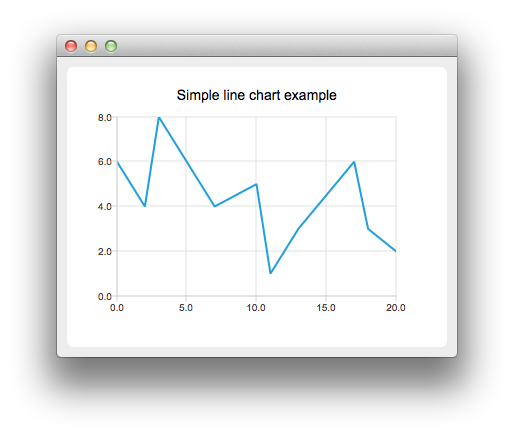# LineChart Example## Running the Example

To run the example from Qt Creator, open the Welcome mode and select the example from Examples. For more information, visit Building and Running an Example.

## Creating Line Charts

To create a line chart, a QLineSeries instance is needed. Let's create one.

`    QLineSeries *series = new QLineSeries();`

Then we add data to the series. We can use the append() member function or use the stream operator.

```    series->append(0, 6);
series->append(2, 4);
series->append(3, 8);
series->append(7, 4);
series->append(10, 5);
*series << QPointF(11, 1) << QPointF(13, 3) << QPointF(17, 6) << QPointF(18, 3) << QPointF(20, 2);```

To present the data on the chart we need a QChart instance. We add the series to it, create the default axes, and set the title of the chart.

```    QChart *chart = new QChart();
chart->legend()->hide();
chart->createDefaultAxes();
chart->setTitle("Simple line chart example");```

Then we create a QChartView object with QChart as a parameter. This way we don't need to create a QGraphicsView scene ourselves. We also set the Antialiasing on to have the rendered lines look nicer.

```    QChartView *chartView = new QChartView(chart);
chartView->setRenderHint(QPainter::Antialiasing);```

The chart is ready to be shown.

```    QMainWindow window;
window.setCentralWidget(chartView);
window.resize(400, 300);
window.show();```

Files: